# Forecasting Time Series with Auto-ArimaPosted by

In this article, I attempt to compare the results of the auto arima function with the ARIMA model we developed in the article Forecasting Time Series with ARIMA (https://www.alldatascience.com/time-series/forecasting-time-series-with-arima/).

I made this attempt to see how it works and what the differences are.The parameters selected by auto-arima are slightly different than the ones selected by me in the other article.
Auto arima has the advantage of attempting to find the best ARIMA parameters by comparing the AIC (Akaike Information Criterion) and BIC (Bayesian Information Criterion) of the tested models, but as demonstrated in this test, it is not always able to do so; the results, as described later, are very similar to those obtained by running the ARIMA algorithm and estimating the parameters “manually,” if not slightly worse.

The first steps in the notebook (data acquisition and cleaning) are the same as in the original article.

European_Countries_Unemployment_Data_Auto_Arima

Let's import the necessary libraries

In :
import pandas as pd
import glob
import numpy as np
import seaborn as sn
import matplotlib.pyplot as plt
from statsmodels.graphics.tsaplots import plot_pacf, plot_acf
from statsmodels.tsa.statespace.sarimax import SARIMAX
from statsmodels.tsa.stattools import pacf


In :
from google.colab import drive
drive.mount('/content/drive')

Drive already mounted at /content/drive; to attempt to forcibly remount, call drive.mount("/content/drive", force_remount=True).


Let's import the data, before doing it I've had to remove the double quotes from the csv file.

In :
data = pd.read_csv('/content/drive/MyDrive/DP_LIVE_10082021082010744.csv', parse_dates=['TIME'], index_col='TIME', header=0,sep=",")

Out:
LOCATION INDICATOR SUBJECT MEASURE FREQUENCY Value Flag Codes
TIME
2000-01-01 EU27_2020 HUR TOT PC_LF M 9.2 NaN
2000-02-01 EU27_2020 HUR TOT PC_LF M 9.2 NaN
2000-03-01 EU27_2020 HUR TOT PC_LF M 9.2 NaN
2000-04-01 EU27_2020 HUR TOT PC_LF M 9.1 NaN
2000-05-01 EU27_2020 HUR TOT PC_LF M 9.1 NaN

Let's remove the unnecessary columns, we are only interested in TIME and Value

In :
data.drop(['LOCATION','INDICATOR','SUBJECT','MEASURE','FREQUENCY','Flag Codes'],axis=1,inplace=True)


Let's plot the data

In :
data.plot(figsize=(15,5))

Out:
<matplotlib.axes._subplots.AxesSubplot at 0x7fe2dd2afc10>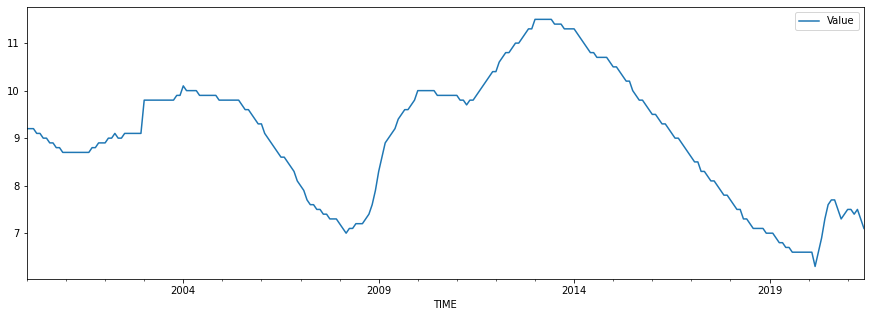In :
#In google colab we should install pmdarima in order to use it.
!pip install pmdarima

Requirement already satisfied: pmdarima in /usr/local/lib/python3.7/dist-packages (1.8.3)
Requirement already satisfied: scipy>=1.3.2 in /usr/local/lib/python3.7/dist-packages (from pmdarima) (1.4.1)
Requirement already satisfied: statsmodels!=0.12.0,>=0.11 in /usr/local/lib/python3.7/dist-packages (from pmdarima) (0.13.0)
Requirement already satisfied: pandas>=0.19 in /usr/local/lib/python3.7/dist-packages (from pmdarima) (1.1.5)
Requirement already satisfied: numpy>=1.19.3 in /usr/local/lib/python3.7/dist-packages (from pmdarima) (1.19.5)
Requirement already satisfied: setuptools!=50.0.0,>=38.6.0 in /usr/local/lib/python3.7/dist-packages (from pmdarima) (57.4.0)
Requirement already satisfied: joblib>=0.11 in /usr/local/lib/python3.7/dist-packages (from pmdarima) (1.0.1)
Requirement already satisfied: scikit-learn>=0.22 in /usr/local/lib/python3.7/dist-packages (from pmdarima) (0.22.2.post1)
Requirement already satisfied: Cython!=0.29.18,>=0.29 in /usr/local/lib/python3.7/dist-packages (from pmdarima) (0.29.24)
Requirement already satisfied: urllib3 in /usr/local/lib/python3.7/dist-packages (from pmdarima) (1.24.3)
Requirement already satisfied: python-dateutil>=2.7.3 in /usr/local/lib/python3.7/dist-packages (from pandas>=0.19->pmdarima) (2.8.2)
Requirement already satisfied: pytz>=2017.2 in /usr/local/lib/python3.7/dist-packages (from pandas>=0.19->pmdarima) (2018.9)
Requirement already satisfied: six>=1.5 in /usr/local/lib/python3.7/dist-packages (from python-dateutil>=2.7.3->pandas>=0.19->pmdarima) (1.15.0)
Requirement already satisfied: patsy>=0.5.2 in /usr/local/lib/python3.7/dist-packages (from statsmodels!=0.12.0,>=0.11->pmdarima) (0.5.2)


Let's try it. First we should split the data into train and test sets.

In :
TEST_SIZE = 36
train, test = data.iloc[:-TEST_SIZE], data.iloc[-TEST_SIZE:]
x_train, x_test = np.array(range(train.shape)), np.array(range(train.shape, data.shape))
train.shape, x_train.shape, test.shape, x_test.shape

Out:
((222, 1), (222,), (36, 1), (36,))
In :
fig, ax = plt.subplots(1, 1, figsize=(15, 5))
ax.plot(x_train, train)
ax.plot(x_test, test)

Out:
[<matplotlib.lines.Line2D at 0x7fe2dc640ed0>]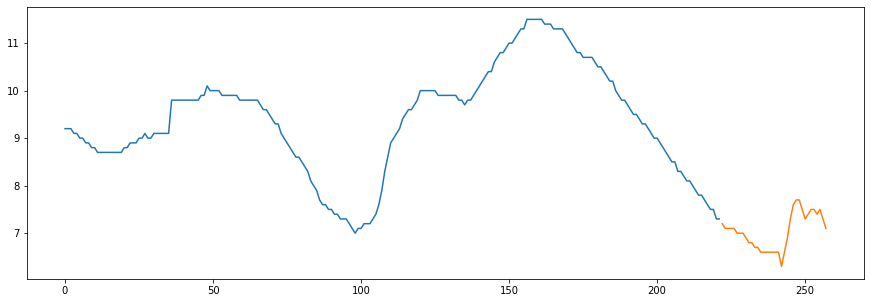In :
from pmdarima.arima import auto_arima


Let's fit the auto_arima model.

In :
model = auto_arima(train, start_p=1, start_q=1,
max_p=5, max_q=5,
m=1,
d=1,
seasonal=False,
start_P=0,
D=None,
trace=True,
error_action='ignore',
suppress_warnings=True,
stepwise=True)

Performing stepwise search to minimize aic
ARIMA(1,1,1)(0,0,0) intercept   : AIC=-456.381, Time=0.20 sec
ARIMA(0,1,0)(0,0,0) intercept   : AIC=-368.929, Time=0.11 sec
ARIMA(1,1,0)(0,0,0) intercept   : AIC=-414.310, Time=0.08 sec
ARIMA(0,1,1)(0,0,0) intercept   : AIC=-392.956, Time=0.11 sec
ARIMA(0,1,0)(0,0,0)             : AIC=-369.426, Time=0.03 sec
ARIMA(2,1,1)(0,0,0) intercept   : AIC=-458.020, Time=0.41 sec
ARIMA(2,1,0)(0,0,0) intercept   : AIC=-449.037, Time=0.22 sec
ARIMA(3,1,1)(0,0,0) intercept   : AIC=-456.422, Time=0.64 sec
ARIMA(2,1,2)(0,0,0) intercept   : AIC=-457.172, Time=0.58 sec
ARIMA(1,1,2)(0,0,0) intercept   : AIC=-457.553, Time=0.52 sec
ARIMA(3,1,0)(0,0,0) intercept   : AIC=-452.103, Time=0.25 sec
ARIMA(3,1,2)(0,0,0) intercept   : AIC=-454.830, Time=0.70 sec
ARIMA(2,1,1)(0,0,0)             : AIC=-459.788, Time=0.21 sec
ARIMA(1,1,1)(0,0,0)             : AIC=-458.155, Time=0.16 sec
ARIMA(2,1,0)(0,0,0)             : AIC=-450.723, Time=0.10 sec
ARIMA(3,1,1)(0,0,0)             : AIC=-458.188, Time=0.30 sec
ARIMA(2,1,2)(0,0,0)             : AIC=-458.941, Time=0.25 sec
ARIMA(1,1,0)(0,0,0)             : AIC=-415.728, Time=0.05 sec
ARIMA(1,1,2)(0,0,0)             : AIC=-459.322, Time=0.21 sec
ARIMA(3,1,0)(0,0,0)             : AIC=-453.835, Time=0.15 sec
ARIMA(3,1,2)(0,0,0)             : AIC=-456.947, Time=0.50 sec

Best model:  ARIMA(2,1,1)(0,0,0)
Total fit time: 5.818 seconds


According to the algorithm the best ARIMA model is ARIMA (2,1,1)

In :
model.summary()

Out:
Dep. Variable: No. Observations: y 222 SARIMAX(2, 1, 1) 233.894 Wed, 06 Oct 2021 -459.788 07:56:45 -446.196 0 -454.300 - 222 opg
coef std err z P>|z| [0.025 0.975] 0.7297 0.108 6.739 0.000 0.518 0.942 0.1793 0.071 2.534 0.011 0.041 0.318 -0.5705 0.102 -5.594 0.000 -0.770 -0.371 0.0070 0.000 34.608 0.000 0.007 0.007
 Ljung-Box (L1) (Q): Jarque-Bera (JB): 0.01 4298.76 0.94 0 0.37 2.83 0 23.85

Warnings:
 Covariance matrix calculated using the outer product of gradients (complex-step).

According to the model summary, the model meets the condition of independence in the residuals (no correlation) because the p-value of the Ljung-Box test (Prob(Q)) is greater than 0.05, so we cannot reject the null hypothesis of independence, but we cannot say that the residual distribution is homoscedastic (constant variance) because the p-value of the Heteroskedasticity test (Prob(H)) is smaller than 0.05.

Let's make the prediction

In :
# Forecast

prediction, confint = model.predict(n_periods=TEST_SIZE, return_conf_int=True)

prediction

Out:
array([7.22732391, 7.17428958, 7.12255592, 7.07529366, 7.03152758,
6.99111462, 6.95377551, 6.91928079, 6.88741293, 6.85797207,
6.83077333, 6.80564597, 6.78243223, 6.76098639, 6.74117379,
6.72287006, 6.7059603 , 6.69033832, 6.67590608, 6.66257295,
6.65025523, 6.63887559, 6.62836259, 6.61865023, 6.60967754,
6.60138818, 6.59373011, 6.58665526, 6.58011921, 6.57408092,
6.5685025 , 6.5633489 , 6.5585878 , 6.55418928, 6.55012574,
6.54637167])
In :
cf= pd.DataFrame(confint)

In :
#Mostramos la gráfica con la predicción de los 2 últimos años en naranja
#sobre la serie real
prediction_series = pd.Series(prediction,index=test.index)
fig, ax = plt.subplots(1, 1, figsize=(15, 5))
ax.plot(data.Value)
ax.plot(prediction_series)
ax.fill_between(prediction_series.index,
cf,
cf,color='grey',alpha=.3)

Out:
<matplotlib.collections.PolyCollection at 0x7fe2cdb4c950>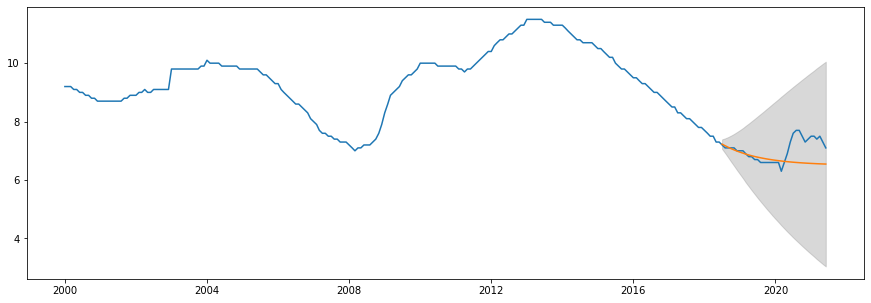In :
model.plot_diagnostics(figsize=(14,10))
plt.show()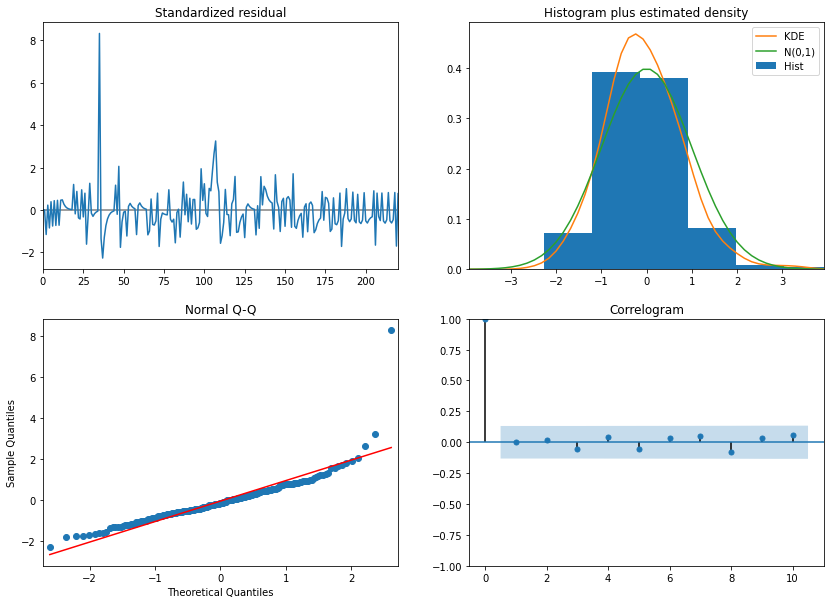We can see from the model plots that the Correlogram does not show any significant correlation in the residuals. The standardized residual plot depicts variance change, while the normal Q-Q plot demonstrates that the residuals do not follow a normal distribution (but this is not a strict requirement to validate the model).

Let's calculate the SMAPE (Symmetric Mean Absolute Error)

In :
def calcsmape(actual, forecast):
return 1/len(actual) * np.sum(2 * np.abs(forecast-actual) / (np.abs(actual) + np.abs(forecast)))

In :
smape=calcsmape(test.Value,prediction)
smape

Out:
0.0531805335780013

Now with 1 year prediction

In :
TEST_SIZE = 12
train, test = data.iloc[:-TEST_SIZE], data.iloc[-TEST_SIZE:]
x_train, x_test = np.array(range(train.shape)), np.array(range(train.shape, data.shape))
train.shape, x_train.shape, test.shape, x_test.shape

Out:
((246, 1), (246,), (12, 1), (12,))
In :
fig, ax = plt.subplots(1, 1, figsize=(15, 5))
ax.plot(x_train, train)
ax.plot(x_test, test)

Out:
[<matplotlib.lines.Line2D at 0x7fe2c05c5d10>]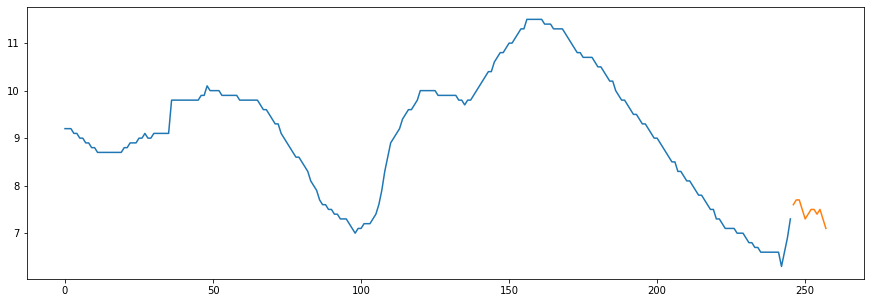Let's fit the new model.

In :
model = auto_arima(train, start_p=1, start_q=1,
max_p=5, max_q=5,
m=1,
d=1,
seasonal=False,
start_P=0,
D=None,
trace=True,
error_action='ignore',
suppress_warnings=True,
stepwise=True)

Performing stepwise search to minimize aic
ARIMA(1,1,1)(0,0,0) intercept   : AIC=-470.207, Time=0.32 sec
ARIMA(0,1,0)(0,0,0) intercept   : AIC=-388.075, Time=0.17 sec
ARIMA(1,1,0)(0,0,0) intercept   : AIC=-434.457, Time=0.07 sec
ARIMA(0,1,1)(0,0,0) intercept   : AIC=-413.125, Time=0.37 sec
ARIMA(0,1,0)(0,0,0)             : AIC=-388.831, Time=0.04 sec
ARIMA(2,1,1)(0,0,0) intercept   : AIC=-469.978, Time=0.38 sec
ARIMA(1,1,2)(0,0,0) intercept   : AIC=-469.613, Time=0.48 sec
ARIMA(0,1,2)(0,0,0) intercept   : AIC=-440.062, Time=0.23 sec
ARIMA(2,1,0)(0,0,0) intercept   : AIC=-465.135, Time=0.27 sec
ARIMA(2,1,2)(0,0,0) intercept   : AIC=-470.017, Time=0.67 sec
ARIMA(1,1,1)(0,0,0)             : AIC=-472.206, Time=0.16 sec
ARIMA(0,1,1)(0,0,0)             : AIC=-414.332, Time=0.17 sec
ARIMA(1,1,0)(0,0,0)             : AIC=-436.122, Time=0.05 sec
ARIMA(2,1,1)(0,0,0)             : AIC=-471.977, Time=0.19 sec
ARIMA(1,1,2)(0,0,0)             : AIC=-471.611, Time=0.20 sec
ARIMA(0,1,2)(0,0,0)             : AIC=-441.574, Time=0.12 sec
ARIMA(2,1,0)(0,0,0)             : AIC=-467.098, Time=0.10 sec
ARIMA(2,1,2)(0,0,0)             : AIC=-472.016, Time=0.32 sec

Best model:  ARIMA(1,1,1)(0,0,0)
Total fit time: 4.331 seconds

In :
model.summary()

Out:
Dep. Variable: No. Observations: y 246 SARIMAX(1, 1, 1) 239.103 Wed, 06 Oct 2021 -472.206 07:56:51 -461.703 0 -467.977 - 246 opg
coef std err z P>|z| [0.025 0.975] 0.9310 0.046 20.226 0.000 0.841 1.021 -0.6716 0.062 -10.771 0.000 -0.794 -0.549 0.0083 0.000 31.761 0.000 0.008 0.009
 Ljung-Box (L1) (Q): Jarque-Bera (JB): 0.6 2419.79 0.44 0 0.84 2.31 0.43 17.69

Warnings:
 Covariance matrix calculated using the outer product of gradients (complex-step).

This time, the p-value of the Heteroskedasticity test is greater than 0.05, indicating that the model explains better the variance in the data. This model outperforms the previous one in terms of dependability. This demonstrates that ARIMA is superior for short-term forecasting.

Let's predict!

In :
prediction, confint = model.predict(n_periods=TEST_SIZE, return_conf_int=True)

prediction

Out:
array([7.46670305, 7.62191067, 7.76641558, 7.90095578, 8.02621844,
8.1428433 , 8.251426  , 8.35252111, 8.44664496, 8.53427827,
8.6158686 , 8.69183267])
In :
cf= pd.DataFrame(confint)

In :
prediction_series = pd.Series(prediction,index=test.index)
fig, ax = plt.subplots(1, 1, figsize=(15, 5))
ax.plot(data.Value)
ax.plot(prediction_series)
ax.fill_between(prediction_series.index,
cf,
cf,color='grey',alpha=.3)

Out:
<matplotlib.collections.PolyCollection at 0x7fe2c32ad890>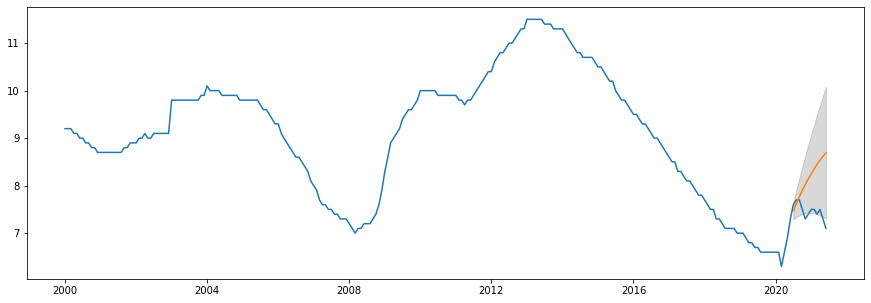In :
model.plot_diagnostics(figsize=(14,10))
plt.show()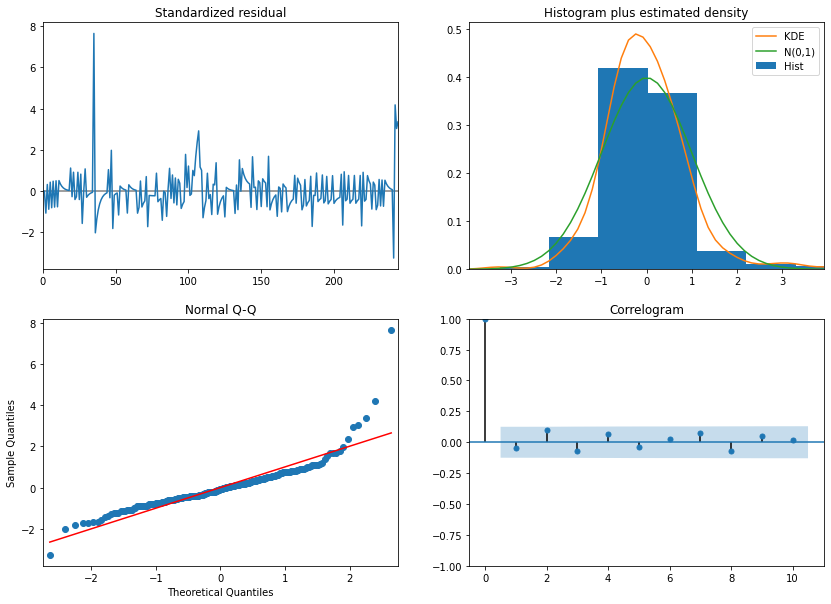Again, the plots show that the residuals are not correlated. The standardized residuals model seems similar to the previous one but as we have seen in the model summary this model is more reliable because we can't reject the null hypothesis of homoscedasticity (constant variance).

In :
smape=calcsmape(test.Value,prediction)
smape

Out:
0.09249388439490931

Conclusion

As we have seen in the notebook the results are slightly different than in the original attempt. The parameters selAs we can see from the notebook, the results differ slightly from the first attempt. The parameters chosen by auto-arima differ. The results are very similar, though slightly worse with the auto-arima model, as evidenced by the SMAPE metric.

Again, the 3-year prediction produces a higher SMAPE value, but the heteroscedasticity test reveals that this model does not account for all of the variance in the data. The 1-year prediction is more reliable because it meets all of the conditions in a valid ARIMA model (non-correlation and constant variance in the residuals).

This has been a really interesting project that has helped me better understand how ARIMA works and how to handle real-world data in order to make a prediction.